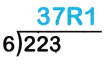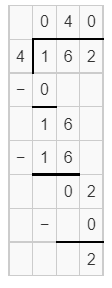# McGraw Hill Math Grade 4 Chapter 6 Lesson 3 Answer Key Dividing a Three-Digit Number

Practice the questions of McGraw Hill Math Grade 4 Answer Key PDF Chapter 6 Lesson 3 Dividing a Three-Digit Number to secure good marks & knowledge in the exams.

## McGraw-Hill Math Grade 4 Answer Key Chapter 6 Lesson 3 Dividing a Three-Digit Number

Divide

Find each quotient and remainder. Not all problems will have remainders.

Question 1.Explanation:
quotient =  37
remainder = 1

Question 2.Explanation:
quotient = 24
remainder = 0

Question 3.Explanation:
quotient = 40
remainder = 2

Question 4.
A large jar contains 321 buttons. Ms. Patel divides the buttons into 3 equal groups. Write the number sentence that shows how Ms. Patel divides the buttons.